Solutions to M362K Practice First Midterm

This exam was originally given on February 27, 1992

Problem 1. Baseball

Two teams (X and Y) play a series of games until one of the teams has won 3 games. (This is commonly called a ``best-of-5'' series, although it is often over in less than 5 games).

a) Explicitly write down the sample space (i.e. list all the possible outcomes).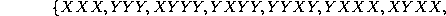XXYX, XXYYX, XXYYY, XYXYX, XYXYY, XYYXX, XYYXY,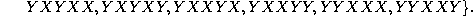Each of the 3-game outcomes has probability 1/8, each of the 4-game outcomes has probability 1/16, and each 5-game outcome has probability 1/32.

b) Let A be the event that team X wins the series, and B be the event that team Y wins the first game. Explicitly write down the set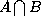.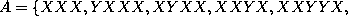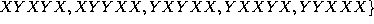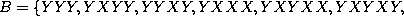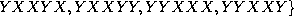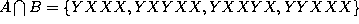.

c) Assuming that each game is independent of the other games, and that the teams are evenly matched, calculate P(A), P(B), and P(A|B).

P(A)=P(B)=1/2 (by symmetry).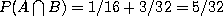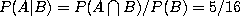.

Problem 2. Politicians.

Suppose that 60% of all politicians are Democrats, while 40% are Republicans. (We'll ignore independents and third-party candidates in this problem). Suppose that among the Democrats 85% are liars and 15% are truthful, while among the Republicans 90% are liars, and 10% are truthful.

(A word about the accuracy of these numbers. You may think that I've grossly underestimated the number of liars, but I'm including local politicians, who run for offices like county dogcatcher. At least some of them are honest.)

a) What is the probability that a randomly chosen politician is truthful?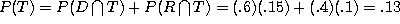b) Given that a certain politician is truthful, what is the probability that he is a Democrat?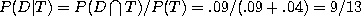Problem 3. Bridge

A bridge hand consists of 13 cards from a standard 52-card deck. You are dealt one hand. Let A be the event that the hand contains exactly 3 aces. Let B be the event that the hand contains exactly 2 kings. Find P(A), P(B), and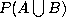. Are A and B independent?

Number of hands is 52 choose 13. Number of hands with 3 aces is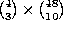. Number of hands with 2 kings is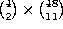. Number of hands with 3 aces and 2 kings is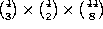. The number of hands with 3 aces OR 2 kings is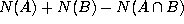.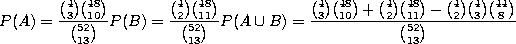Problem 4. Continuous distributions:

The amount of time it takes a driver to react to a changing stoplight varies from driver to driver, and is (roughly) described by the continuous probability function of the form: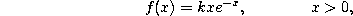where k is a constant and x is measured in seconds.

a) Find the constant k. (Hint: integrate by parts)

k=1, since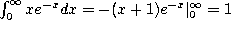.

b) Find the probability that a driver takes more than 1 second to react.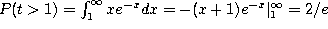.

Extra credit: Flipping coins:

Players A and B take turns flipping a fair coin, with A going first. The first player to toss a head wins. What is the probability of A winning?

A winning is the set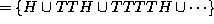. The probability of that is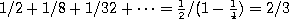.